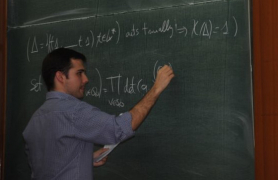## 30/11/2021, Tuesday, 16:30–17:30 Europe/Lisbon — OnlineAlfonso Zamora, Polytechnic University of Madrid
E-polynomials and geometry of character varieties

Quasi-projective complex algebraic varieties (not necessarily smooth or compact) carry, following Deligne, mixed Hodge structures whose numerical information is encoded in the $E$-polinomial, which provides substantial geometrical (topological plus complex) information about these varieties; important examples being character varieties. Let $G$ be a complex algebraic group and let $\mathcal{X}_{\Gamma}G$ be the $G$-character variety of a finitely presented group $\Gamma$. Exploring a new path between the previously known arithmetical and geometrical methods to compute $E$-polynomials we provide a concrete relation, in terms of plethystic functions, stratifications of the character variety as an affine GIT quotient and combinatorics, between the generating series for $E$-polynomials of $\mathcal{X}_{\Gamma}G$ for $G=GL(n,\mathbb{C})$ and the series of the subset of irreducible representation conjugacy classes $\mathcal{X}_{\Gamma}^{irr}G$. This yields explicit (stratified) expressions for the $E$-polynomials of several groups $\Gamma$, for low values of $n$. For the case $\Gamma=F_{r}$, the free group of rank $r$, we prove the equality of the $E$-polynomials for $\mathcal{X}_{r}SL_{n}$ and $\mathcal{X}_{r}PGL_{n}$, for any $n,r\in\mathbb{N}$. This settles a conjecture of Lawton-Muñoz and provides evidence on topological mirror symmetry conjectures for Langlands dual groups.

These results are joint work with Carlos Florentino and Azizeh Nozad.

### Video